# Multiply rational numbersPage 1

#### WATCH ALL SLIDES

Slide 1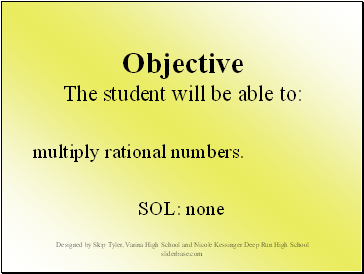multiply rational numbers.

SOL: none

Objective The student will be able to:

Designed by Skip Tyler, Varina High School and Nicole Kessinger Deep Run High School

sliderbase.com

Slide 2## Multiplying Rules

1) If the numbers have the same signs then the product is positive.

(-7) • (-4) = 28

2) If the numbers have different signs then the product is negative.

(-7) • 4 = -28

sliderbase.com

Slide 3Examples

1) (3x)(-8y)

Write both numbers as a fraction.

Cross-cancel if possible.

Multiplying fractions:

top # • top #

Bottom # • bottom#

-24xy

sliderbase.com

Slide 4When multiplying two negative numbers, the product is negative.

True

False

sliderbase.com

Slide 5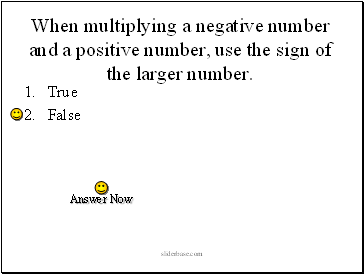When multiplying a negative number and a positive number, use the sign of the larger number.

True

False

sliderbase.com

Slide 6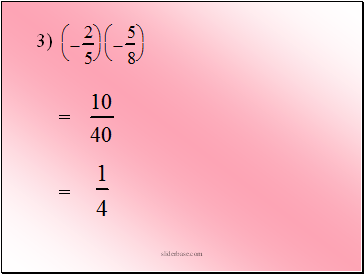sliderbase.com

Slide 7Multiply: (-3)(4)(-2)(-3)

72

-72

36

-36

sliderbase.com

Slide 8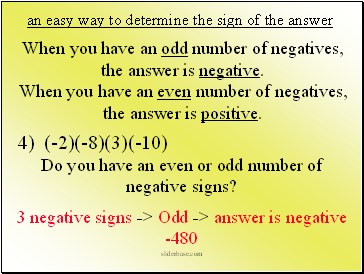an easy way to determine the sign of the answer

3 negative signs -> Odd -> answer is negative

-480

When you have an odd number of negatives, the answer is negative.

When you have an even number of negatives, the answer is positive.

4) (-2)(-8)(3)(-10)

Do you have an even or odd number of negative signs?

sliderbase.com

Slide 9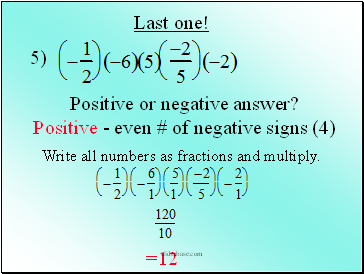Positive - even # of negative signs (4)

Write all numbers as fractions and multiply.

=12

sliderbase.com

Slide 10What is the sign of the product of (-3)(-4)(-5)(0)(-1)(-6)(-91)?

Positive

Negative

Zero

Huh?

sliderbase.com